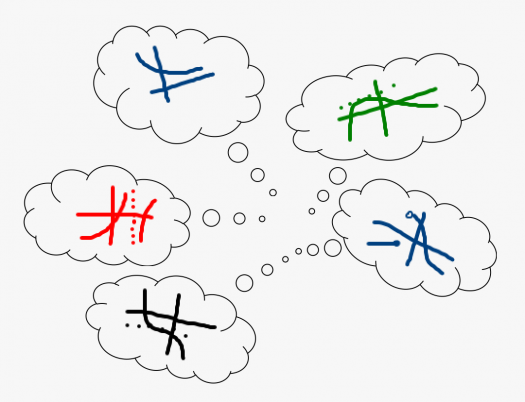# Connections Between Function Features And Limits

5 Questions | Total Attempts: 441SettingsWhat does different limits information tell us about the features of a function?

• 1.
Select all the places to look for extreme points (maxima, minima):
• A.

Where the instantaneous rate of change is zero

• B.

Where the instantaneous rate of change does not exist

• C.

At the endpoints of the interval of interest

• D.

Where the function value is zero

• 2.
Which of the following must be true if a function f(x) does not have a minimum on an interval [a,b].
• A.

F(x) must approach negative infinity somewhere in the interval [a,b]

• B.

F(x) must have a discontinuity on [a,b]

• C.

F(a) must be greater than f(b)

• D.

F(x) must have a maximum on the interval [a,b]

• 3.
Which existence theorem allows use to use guess-and-check to solve equations which can be written in the form F(x) = 0 for a continuous function F?
• A.

The Intermediate Value Theorem

• B.

The Mean Value Theorem

• C.

The Extreme Value Theorem

• D.

Rolle's Theorem

• 4.
Select all the correct statements about extreme points of the function in the graph.
• A.

The absolute minimum value is 0.

• B.

The absolute maximum value is 14/3.

• C.

There is a relative minimum between x=–5 and x=–4.

• D.

There is one point which is a relative maximum where the instantaneous rate of change is zero.

• E.

The instantaneous rate of change does not exist at x=–3 and there is a relative maximum there.

• F.

(1,1) is a relative maximum point.

• G.

The left end point is a relative maximum.Back to top